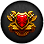# Forums

## Pet Pre-Insurance.

Quick find code: 322-323-363-65637914

of 36

### insaneDemNS

Posts: 1,011Mithril Posts by user Forum Profile RuneMetrics Profile
Providential said:
You're welcome.Sorry for not replying you in-game due to some mandatory business I have to attend on the spot, when I'm back, you have logged.Regardless, do enjoy your vacation and trip!Miaow.No worries! Yes.. the trip. It's coming soon. So please do keep an eye on the thread until.. the 17th lol.

@Lavine
DOOM

Rider said:
Support

Woo! Woof! Appreciate it.

29-Sep-2015 07:49:19

### insaneDemNS

Posts: 1,011Mithril Posts by user Forum Profile RuneMetrics Profile
Providential said:
Will do. You can count on me!Up.
Yus! Only days away.

Relmer1 said:
I feel that this would take away some of the achievement in owning a wilderness boss.
A fair point. :E

04-Oct-2015 06:56:32

Sep2018

### Providential

Posts: 1,096Mithril Posts by user Forum Profile RuneMetrics Profile
^
^
^
^
^
^
^
^
^
^
^
^
^
^
^
^^
^
^
^
^
^
^
^^
^
^
^
^
^
^
^^
^
^
^
^
^
^
^^
^
^
^
^
^
^
^^
^
^
^
^
^
^
^^
^
^
^
^
^
^
^^
^
^
^
^
^
^
^^
^
^
^
^
^
^
^^
^
^
^
^
^
^
^^
^
^
^
^
^
^
^^
^
^
^
^
^
^
^^
^
^
^
^
^
^
^^
^
^
^
^
^
^
^^
^
^
^
^
^
^
^^
^
^
^
^
^
^
^^
^
^
^
^
^
^
^^
^
^
^
^
^
^
^^
^
^
^
^
^
^
^^
^
^
^
^
^
^
^^
^
^
^
^
^
^
^^
^
^
^
^
^
^
^^
^
^
^
^
^
^
^^
^
^
^
^
^
^
^^
^
^
^
^
^
^
^^
^
^
^
^
^
^
^^
^
^
^
^
^
^
^^
^
^
^
^
^
^
^^
^
^
^
^
^
^
^^
^
^
^
^
^
^
^^
^
^
^
^
^
^
^^
^
^
^
^
^
^
^^
^
^
^
^
^
^
^^
^
^
^
^
^
^
^^
^
^
^
^
^
^
^^
^
^
^
^
^
^
^^
^
^
^
^
^
^
^^
^
^
^
^
^
^
^^
^
^
^
^
^
^
^^
^
^
^
^
^
^
^^
^
^
^
^
^
^
^^
^
^
^
^
^
^
^^
^
^
^
^
^
^
^^
^
^
^
^
^
^
^^
^
^
^
^
^
^
^^
^
^
^
^
^
^
^^
^
^
^
^
^
^
^^
^
^
^
^
^
^
^^
^
^
^
^
^
^
^^
^
^
^
^
^
^
^^
^
^
^
^
^
^
^^
^
^
^
^
^
^
^^
^
^
^
^
^
^
^^
^
^
^
^
^
^
^^
^
^
^
^
^
^
^^
^
^
^
^
^
^
^^
^
^
^
^
^
^
^^
^
^
^
^
^
^
^^
^
^
^
^
^
^
^^
^
^
^
^
^
^
^^
^
^
^
^
^
^
^^
^
^
^
^
^
^
^^
^
^
^
^
^
^
^^
^
^
^
^
^
^
^^
^
^
^
^
^
^
^^
^
^
^
^
^
^
^^
^
^
^
^
^
^
^^
^
^
^
^
^
^
^^
^
^
^
^
^
^
^^
^
^
^
^
^
^
^^
^
^
^
^
^
^
^^
^
^
^
^
^
^
^^
^
^
^
^
^
^
^^
^
^
^
^
^
^
^^
^
^
^
^
^
^
^^
^
^
^
^
^
^
^^
^
^
^
^
^
^
^^
^
^
^
^
^
^
^^
^
^
^
^
^
^
^^
^
^
^
^
^
^
^^
^
^
^
^
^
^
^^
^
^
^
^
^
^
^^
^
^
^
^
^
^
^^
^
^
^
^
^
^
^^
^
^
^
^
^
^Up.
C(h)vt(k)y

04-Oct-2015 18:32:39 - Last edited on 04-Oct-2015 18:32:58 by Providential

### insaneDemNS

Posts: 1,011Mithril Posts by user Forum Profile RuneMetrics Profile
Lol. Very nicely done, friend.

*scrolls down*

*keeps scrolling*

*picks up the pace*

*moves mouse downwards even more*

*GAHHH*

Anyway! I'll be leaving in about 12 hours, so you'll be on the clock soon! I'll try and get a couple of bumps in myself until then. ^^ Thanks for your dedication! Oh and if at any time you see a post made by another user, you're more than welcome to respond (although not required!).

Thanks again.

Woof.

06-Oct-2015 13:46:27 - Last edited on 06-Oct-2015 13:46:58 by insaneDemNS